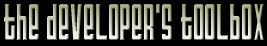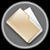This section of the archives stores flipcode's complete Developer Toolbox collection, featuring a variety of mini-articles and source code contributions from our readers.OpenGL/Direct3D Projection Matrix   Submitted byHere is a projection matrix that I have worked out for use OpenGL. I am very new to both OpenGL and D3D. From the first impression I like OpenGL more but what is really a nuisance of OpenGL is that negative z coordinates point into the distance. I don't like the matrix that is produced by glFrustum(...) because I want to have the far plane at infinity. Here is the projection matrix that I use (it's working well both with Microsoft OpenGL and the ICD Driver of my Voodoo Card):

 ```- far plane at infinity - positive z with increasing depthfloat matrix[] = { focus, 0, 0, 0, 0, 4*focus/3, 0, 0, 0, 0, 65535f/65536f, 1, 0, 0, -near, 0 };glMatrixMode( GL_PROJECTION ); glLoadMatrixf( &matrix ); ```

focus
is the focal length of the field of view. It turns out that it is the multiple of screen widths that correspond to a view angle of 90ÂÂ°. If focus is = 1, then from left screen edge to right screen edge is 90ÂÂ°. If focus = 2 then 90ÂÂ° view angle would project onto 2 screen widths, etc. I am multiplying with 4/3 for the y coordinate to get correct aspect ratio.

65535f/65536f
The specification of the transform-pipeline says that after multiplication with the projection matrix, the x, y, and z coordinate are divided by the w coordinate, the resulting x and y are the screen coordinates and the resulting z coordinate goes into the depth buffer of the video card. So if zp were the z coordinate in projection space, and zn the z coordinate in normalized device space, then my matrix does this:

 ` zn = ( 65535/65536 * zp - near ) / zp `

So when zp < near, then zn < 0, so near is the near clipping plane. But if zp = infinity then zn = 65535/65536 (0.9999etc in 16 bits), the highest value that can be written into a 16 bit the depth buffer. Is important that zn doesn't get 1 because that would get clipped by OpenGL.

The mapping of zp -> zn can be controlled with the near parameter. If you need more precision in the front, make the near clipping plane closer, thus you are loosing precision in the distance, but the far clipping plane remains at infinity.

If you have more than 16 bits depth buffer I would advise you use 2097151/2097152. This is 0.9999etc in 21 bits which is the precision of the mantissa of a floating point number. Don't use 4294967295/4294967296 (32 bits) because this could get converted to 1 in floating point.

Exactly the same matrix works with Direct3D, in exactly the same way

 ```D3DMATRIX matrix = D3DMATRIX( focus, 0, 0, 0, 0, 4*focus/3, 0, 0, 0, 0, 65535f/65536f, 1, 0, 0, -near, 0 Device-SetTransform( D3DTRANSFORMSTATE_PROJECTION, &matrix ); ```

The D3DMATRIX is row major instead of OpenGL matrix column-major, but the ordering of the previous float[]-array appears transposed on the screen, so they both look the identical.

Now comes the last: If you use this matrix you must change the depth test function to "less-than".

 ```in OpenGL glDepthFunc( GL_LESS );in Direct3D: Device-SetRenderState( D3DRENDERSTATE_ZFUNC, D3DCMP_LESS ); ```

have fun
chris

The zip file viewer built into the Developer Toolbox made use of the zlib library, as well as the zlibdll source additions.